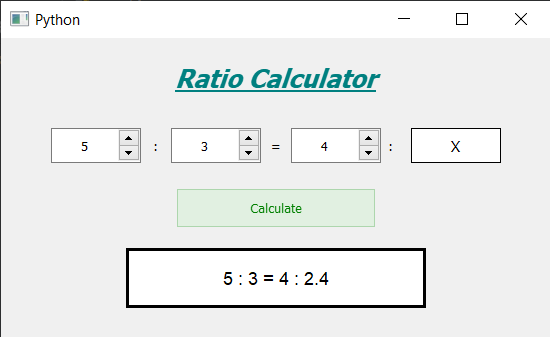Ratio Calculator using PyQt5

• Last Updated : 10 Jul, 2020

In this article we will see how we can create a ratio calculator using PyQt5. Ratio is a contrast, which exists between two particular numbers, is defined as ratio. This ratio calculator is developed to compute this contrast and find out the relationship between these numbers. Below is how the calculator will lookPyQt5 is cross-platform GUI toolkit, a set of python bindings for Qt v5. One can develop an interactive desktop application with so much ease because of the tools and simplicity provided by this library. Below is the command to install the PyQt5

pip install PyQt5

Concept :
User has to select a ratio then enter another number for which calculator will find the corresponding ratio value, below is the formula used

A / B = C / X

Here A and B are the selected ratio and C is the entered number for which we have to find corresponding value of the ratio

GUI Implementation Steps :
1. Create a heading label that display the calculator name
2. Create three spin box for getting ‘A’, ‘B’ and ‘C’ values
3. Create labels between spin boxes for ratio and equal to symbol and label for showing ‘X’ value
4. Create push button for calculate the ratio value
5. Create a label to show the calculated value

Back-End Implementation :
1. Set range to each of the spin box with minimum value equal to 1 so that user can’t enter 0 as input
2. Set various properties like alignment, geometry to each of the widget in the window
3. Add color effect to the push button
4. Add action tot he push button when it get clicked
5. Inside the push button action calculate the value of ‘X’ according to the values entered by user
6. Remove decimal values if it is a whole number
7. Show the output with the help of result label

Below is the implementation